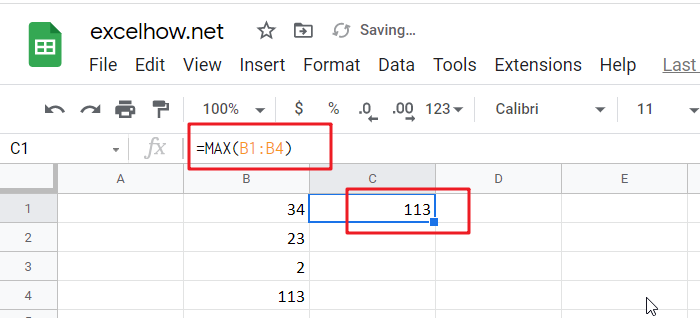# ExcelHow

This post will guide you how to use Google Sheets MAX function with syntax and examples in Google Spreadsheet.

## Description

The Google Sheets MAX function returns the largest numeric value from the numbers that you provided. Or returns the largest value in the array.

The MAX function is a build-in function in Google Sheets and it is categorized as a Statistical Function.

## Syntax

The syntax of the MAX function is as below:

`= MAX(num1,[num2,…numn])`

Where the MAX function arguments are:

• num1 -This is a required argument. A number, or a reference to a number or a range that contains numeric values
• Num2,…Numn – This is an optional argument.  A number, or a reference to a number or a range that contains numeric values

Note:

• The num1 arguments can be numbers, arrays, defined names or references that contain numeric values.
• IF the arguments contains non numeric value, it returns 0.
• The value returned by the MAX function is still a numeric value.
• If the argument is an array or reference, only numbers in that array are used. Empty string, logical values or text of numbers are ignored.

## Google Sheets MAX Function Examples

The below examples will show you how to use Google Sheets MAX Function to get the largest value form the numbers in a range.

#1 To get the largest value from a range B1:B4, just using the following Google Sheets formula:

`=MAX(B1:B4)`